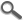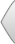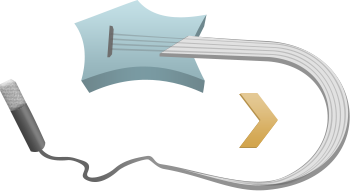• Artists: 53
• Albums: 118
• Songs:  316
• Art:  53
• Alb: 118
• Sng: 316A B C D E F G H I J K L M N O P Q R S T U V W X Y Z #
 A B C D E F G H I J K L M N
 O P Q R S T U V W X Y Z #A . . . . . . Z
A B C D
E F G H
I J K L
M N O P Q
R S T U V
W X Y Z #
0:00 / 4:3
Music:Play!
Guitareoke
preferences - open
font typeface

### Lyrics and Chords

```Intro:
C | G | Am | F | C | G | F  C/E Dm | C

Verse 1:
C              G
When I find myself in times of trouble
Am          F
Mother Mary comes to me
C                G              F  C/E Dm C
Speaking words of wisdom, let it be

C              G
And in my hour of darkness she is
Am                F
Standing right in front of me
C                G              F  C/E Dm C
Speaking words of wisdom, let it be

Chorus:
Am         G          F          C
Let it be, let it be, let it be, let it be
G              F  C/E Dm C
Whisper words of wisdom, let it be

Verse 2:
C              G
And when the broken-hearted people
Am            F
Living in the world agree
C              G               F  C/E Dm C
There will be an answer, let it be

C                 G
For though they may be parted there is
Am                  F
Still a chance that they will see
C              G               F  C/E Dm C
There will be an answer, let it be

Chorus:
Am         G          F          C
Let it be, let it be, let it be, let it be
G           F  C/E Dm C
There will be an answer, let it be

Am         G          F          C
Let it be, let it be, let it be, let it be
G              F  C/E Dm C
Whisper words of wisdom, let it be

Instrumental:
F | C | G F | C/E  - x2
C | G | Am | F | C | G | F | C  - x2

Chorus:
Am         G          F          C
Let it be, let it be, let it be, let it be
G              F C
Whisper words of wisdom, let it be-e

Verse 3:
C                 G
And when the night is cloudy, there is
Am                 F
Still a light that shines on me
C            G              F  C/E Dm C
Shine until tomorrow, let it be

C              G
I wake up to the sound of music
Am          F
Mother Mary comes to me
C                G              F C
Speaking words of wisdom, let it be-e

Chorus:
Am         G          F          C
Let it be, let it be, let it be, let it be
G               F C
There will be an answer, let it be-e

Am         G          F          C
Let it be, let it be, let it be, let it be
G               F C
There will be an answer, let it be-e

Am         G          F          C
Let it be, let it be, let it be, let it be
G              F C
Whisper words of wisdom, let it be-e

Coda:
F | C | G F | C/E
```

key
C
original
key
C
C# B
transpose
capo
on
0 fret
not in use
C
C#
D
D#
E
F
F#
G
G#
A
A#
B